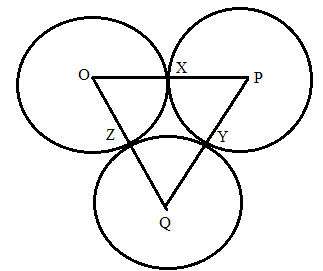Chapter 6.3, Problem 41EElementary Geometry For College St...

7th Edition
Alexander + 2 others
ISBN: 9781337614085

Solutions

Chapter
SectionElementary Geometry For College St...

7th Edition
Alexander + 2 others
ISBN: 9781337614085
Textbook Problem

Circles O, P, and Q are tangent (see Exercise 40) at points X, Y, and Z. being as specific as possible, explain what type of triangle Δ P Q O is if:a) O X = 3 , P Y = 4 , Q Z = 1 b) O X = 2 , P Y = 2 , Q Z = 2 .To determine

a)

To find:

To explain what type of triangle ΔPQO is if:

OX=3,PY=4,QZ=1

Explanation

Given that, circles O, P, and Q are tangent (as shown) at points X, Y, and Z.

Then the value of OX=3,PY=4,QZ=1.

The diagrammatic representation is given below,

To find the measures of the sides of the triangle ΔPQO is given below,

OP=OX+PXPQ=PY+QYOQ=QZ+OZ

We know that OX=OZ,PX=PY,QY=QZ since radius of the circle is same for all points on the circle.

Substitute the value of OX=3,PY=4,QZ=1 and OX=OZ,PX=PY,QY=QZ to get the following,

OP

To determine

b)

To find:

To explain what type of triangle ΔPQO is if:

OX=2,PY=2,QZ=2

Still sussing out bartleby?

Check out a sample textbook solution.

See a sample solution

The Solution to Your Study Problems

Bartleby provides explanations to thousands of textbook problems written by our experts, many with advanced degrees!

Get Started

Solve the equations in Exercises 126. (x2+1)x+1(x+1)3=0

Finite Mathematics and Applied Calculus (MindTap Course List)

Find the maximum revenue R (in dollars) given that 12(2R 320) 4(3R + 240)

Applied Calculus for the Managerial, Life, and Social Sciences: A Brief Approach

A fair die is rolled four times. Calculate the probability obtaining exactly two 6s.

Finite Mathematics for the Managerial, Life, and Social Sciences

Find for defined implicity by .

Study Guide for Stewart's Multivariable Calculus, 8th

Evaluate dx using the Fundamental Theorem of Calculus. 6 12 18 24

Study Guide for Stewart's Single Variable Calculus: Early Transcendentals, 8th